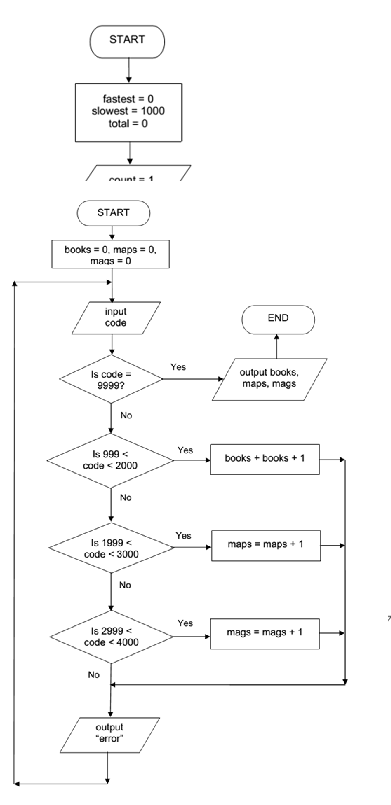Algorithm that inputs the codes for all items in stock, Data Structure & Algorithms

Assignment Help:

A shop sells books, magazines and maps. Every item is identified by a unique 4 - digit code. All books have a code which starts with 1, all maps have a code starting with 2 and all magazines have a code which starts with 3. The code 9999 is used to end algorithm.

Write an algorithm in form of a flowchart that inputs the codes for all items in stock and outputs number of books, number of magazines and number of maps in stock. Include any validation checks needed.Compound interest, Write the algorithm for compound interest

Write the algorithm for compound interest

State the painter algorithm, Painter's Algorithm As the name suggests, ...

Painter's Algorithm As the name suggests, the algorithm follows the standard practice of a painter, who  would paint the background (such as a backdrop) first, then the major d

The data structure needed for Breadth First Traversal on a graph is Queue

Adjacency list representation An Adjacency list representation of Graph G = {V, E} contains an array of adjacency lists mentioned by adj of V list. For each of the vertex u?V,

Logic circuits, the voltage wave forms are applied at the inputs of an EX-O...

the voltage wave forms are applied at the inputs of an EX-OR gate. determine the output wave form

What is an algorithm, What is an algorithm?  What are the characteristics o...

What is an algorithm?  What are the characteristics of a good algorithm? An algorithm is "a step-by-step process for accomplishing some task'' An algorithm can be given in many

Explain in detail about the abstract data type, Abstract data type The ...

Abstract data type The thing which makes an abstract data type abstract is that its carrier set and its operations are mathematical entities, like geometric objects or numbers;

Compare and contrast various sorting techniques, Q. Compare and contrast va...

Q. Compare and contrast various sorting techniques or methods with respect to the memory space and the computing time.

Enumerate about the concept of container, Enumerate about the concept of co...

Enumerate about the concept of container A Container can have a size() operation. We can also ask (somewhat redundantly) whether a Container is empty. And even though a Contain

Multiple Queues in a single dimension array, Implement multiple queues in a...

Implement multiple queues in a single dimensional array. Write algorithms for various queue operations for them.